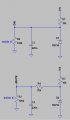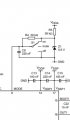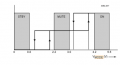# How to take the internal circuitry of an IC into consideration when running simulation?

#### Yami

Joined Jan 18, 2016
282
Hi guys,
Random question, but it's been something that I have been wondering for sometime.
I been trying to simulate the control circuitry for the 'mode' control function of the TDA8924 amplifier chip. I have to assume a value for the 'load' of that particular pin. In this case I use a 100K resistor. This is the only way to figure out what the voltage at the pin would be due to the external components.
So my question is that is there a certain value I should be using for this pin 'load'? Different values for different kind of pins?Maybe a hint given on the datasheet? I can think of other instances where I might encounter the same issue, with different ICs I mean.
Thanks in advance for the help#### ronsimpson

Joined Oct 7, 2019
684
I think there is an error in the data sheet. 'Max voltage on mode pin is 5.5V' One diagram shows a 5.6V Zener I think it should be a 5.1V Zener.
It is clear that when the switch is at "off" the voltage is 0V.
When the switch is in "on" the voltage is the Zener voltage. I think 5.1V but maybe 5.6V which is over spec.
In "mute" mode the voltage should be about 2.6V +/-0.4V.
Assume Zener=5.0V for the sake of round numbers. R4 makes the top half of a voltage divider. Likely in side the IC there is a second 39K to ground. The two 39K resistor will cause of voltage of 1/2 Zener voltage. 5.0V/2=2.5V.I think the resistor inside the IC is 39k not 100k.

•Yami

#### Yami

Joined Jan 18, 2016
282
I think there is an error in the data sheet. 'Max voltage on mode pin is 5.5V' One diagram shows a 5.6V Zener I think it should be a 5.1V Zener.
It is clear that when the switch is at "off" the voltage is 0V.
When the switch is in "on" the voltage is the Zener voltage. I think 5.1V but maybe 5.6V which is over spec.
In "mute" mode the voltage should be about 2.6V +/-0.4V.
Assume Zener=5.0V for the sake of round numbers. R4 makes the top half of a voltage divider. Likely in side the IC there is a second 39K to ground. The two 39K resistor will cause of voltage of 1/2 Zener voltage. 5.0V/2=2.5V.
View attachment 207453
I think the resistor inside the IC is 39k not 100k.
Thanks @ronsimpson, I didn't mean only this instance. But in general. This was what I was messing around today. Can I ask though how you deduced that its a 39K resistor inside the IC.

#### ronsimpson

Joined Oct 7, 2019
684
I tried to explain that "mute" is defined by 2.6V+/-0.4V.
R4=39k. All this we know.
What we need to find is what resistor, along with a R4=39k, will take 5.1V and reduce it to 2.6V. (voltage divider formula)

•Yami

#### Alec_t

Joined Sep 17, 2013
11,324
But in general.
There is no 'in general'. Different ICs have different internal components.

•Yami